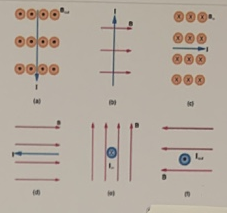# Problem: Consider the magnetic fields and currents depicted in the figure:What is the direction of the magnetic force on the current in (a)? current in (b)?  current in (c)?  current in (d)? current in (e)?

###### FREE Expert Solution

Right-hand rule:

Thumb points in the direction of the magnetic force.

Fingers point in the direction of the field.

Palm points in the direction of the conventional current.

96% (432 ratings)###### Problem Details

Consider the magnetic fields and currents depicted in the figure:What is the direction of the magnetic force on the current in (a)? current in (b)?  current in (c)?  current in (d)? current in (e)?